### Home > CAAC > Chapter 2 > Lesson 2.1.7 > Problem2-70

2-70.
1. Examine the tile pattern at below. Homework Help ✎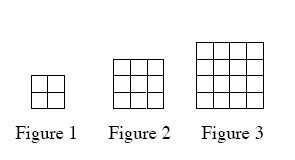1. On graph paper, draw Figures 4 and 5.

2. What would Figure 10 look like? How many tiles would it have? What about Figure 100?

3. Cami has a different tile pattern. She decided to represent the number of tiles of her pattern in a table, as shown below. Can you use the table to predict how many tiles would be in Figure 5 of her tile pattern? How many tiles would Figure 8 have? Explain how you know.

 Figure Number 1 2 3 4 Number of Tiles 5 9 13 17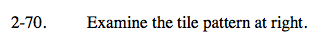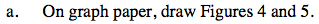Figure 1 is a 2-by-2 square
Figure 2 is a 3-by-3 square
Figure 3 is a 4-by-4 square.
That means Figure x should be a (x + 1)-by-(x + 1) square.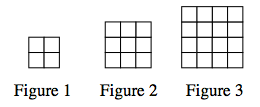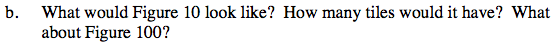Refer to part (a)'s hint.

Figure 10: An 11-by-11 square that would have 121 tiles.
Can you use the pattern to find what Figure 100 would look like?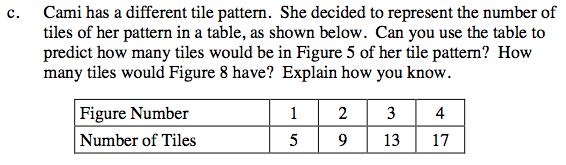Find the pattern in the chart.